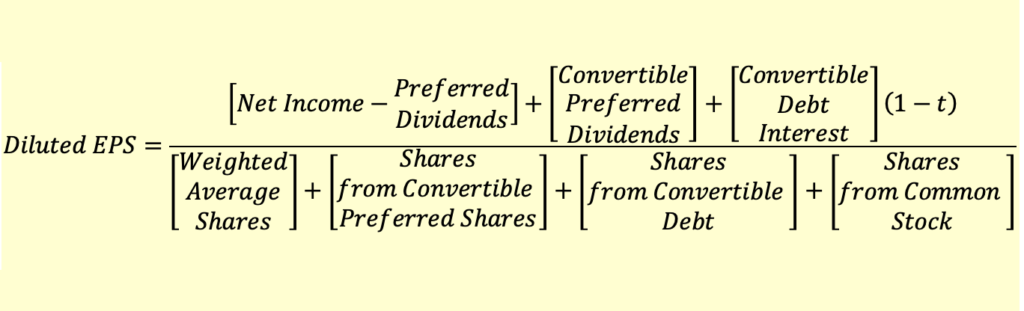# Calculation of Diluted EPS (Convertible Preferred Stock)

We have the following data for a company:

• Net income= $12,000 • Preferred dividend=$2,000
• Weighted Average Shares Outstanding= 10,000
• Convertible Preferred Stock Outstanding = 1,000

Case A: Each share of preferred stock is to be converted into four shares of common stock

Basic EPS = (12,000 – 2,000)/10,000 = 1

The convertible preferred stock should be included in the calculation of diluted EPS only if it is dilutive. To check if it is dilutive, we will divide the preferred dividend by the no. of common shares created from the preferred stock. If this is less that basic EPS, the security is dilutive.

In this example: $2,000/4,000 = 0.5 which is less than basic EPS of 1. Therefore the convertible preferred stock is dilutive and must be included in the calculation of diluted EPS. Diluted EPS = 12,000/(10,000+4,000) =$0.857

Note that in the numerator we did not subtract the preferred dividend as the preferred stock is assumed to be converted into common stock.

Case B: Each share of preferred stock is to be converted into one shares of common stock

Impact of the assumed conversion: $2,000/1,000 shares =$2 > Basic EPS

This security is anti-dilutive and should NOT be considered in the computation of DEPS.

The generalized formula for diluted EPS is presented below: